KING FAHD UNIVERSITY OF PETROLUEM AND MINERALS

Dammam Community College – Prep-Year Math Program

Math 011 - Term 031

Worksheet #1 (P1)

Q1) Which of the following statement is TRUE and which is FALSE .State the

reason .

a)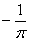is the multiplicative inverse of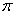b)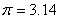c) 0, 1, 2, 3, 4, … are positive integers

d) Every integer is either prime or composite

e) Every rational number has a multiplicative inverse

f) The set of irrational numbers is not closed under addition and multiplication .

g) 1 is the only positive integer that is neither prime nor composite

h) 1.7 +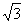is a rational number

i) The set { -1,0,1} is closed under addition

j) The set { -1,0,1} is closed under multiplication

k) 501 is a prime number

l) Every prime number is an odd number

m) All even numbers are composite numbers

n) If  A=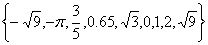,then A has 7 rational numbers

o)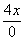is a real number

p) If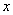is a real number, then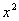is a positive number

q)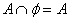for any set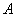r) The sum of two composite numbers is a composite number

s) All integers are natural numbers

t) Every real number is either rational or irrational number

u) 1 is the only natural  positive number that is neither prime nor composite

v) 1 is the only integer  that is neither prime nor composite

w)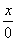is the multiplicative inverse of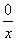x) The operation of division of real numbers is comutative

z) The multiplicative inverse of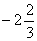is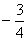Q2)  Name the property of real number or the property of equality of the following :

1)      (a + b ) + c = c + ( a + b )

2)      (4.x).y = y.(4.x)

3)      (4----.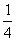)z = 1.z

4)      3 + (-3) = 0

5)      1.z = 1.z

6)      3(x+4) = 3x+12

7)      a(bc) = a(bc)

8)      1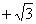is a real number

9)      If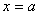and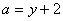, then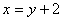10)   If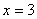and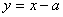, then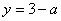Q3) Let A=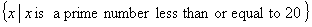B=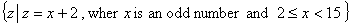.

1-     List all elements of A and B

2-     Find A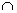B